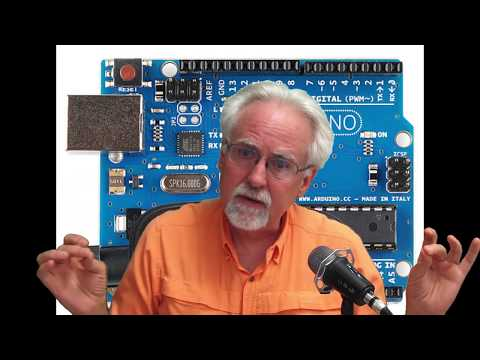# Arduino Tutorial 8: Understanding Pulse Width Modulation (PWM) and the Arduino Analog Write CommandIn Lesson 7, we learned how we can get in-between voltages from the Arduino pins using the analogWrite command. Actually, this command only approximates analog voltages, and does not produce actual analog signals. It works by quickly turning the voltage to the pin on and off. For example, if you ask for 2.5 volts, it will quickly switch the pin on, with it on 50% of the time and off 50% of the time. Similarly, if you asked for 1 volt, it is really switching 5 volts on and off quickly. For this case, it would be on 20% of the time and off 80% of the time. This technique is called Pulse Width Modulation. In this video we show you the actual waveforms coming from the analogWrite command on an oscilloscope. If you want to follow this lesson at home, you can get the Arduino kit we are using HERE.